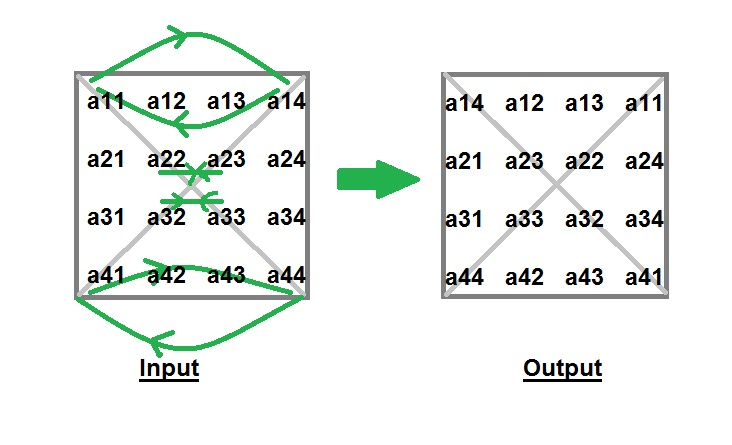GeeksforGeeks App
Open AppBrowser
Continue

# C Program for Program to Interchange Diagonals of Matrix

Given a square matrix of order n*n, you have to interchange the elements of both diagonals.
Examples :

```Input : matrix[][] = {1, 2, 3,
4, 5, 6,
7, 8, 9}
Output : matrix[][] = {3, 2, 1,
4, 5, 6,
9, 8, 7}

Input : matrix[][] = {4,  2,  3,  1,
5,  7,  6,  8,
9, 11, 10, 12,
16, 14, 15, 13}
Output : matrix[][] = {1,  2,  3,  4,
5,  6,  7,  8,
9, 10, 11, 12,
11, 14, 15, 16}```

Explanation : Idea behind interchanging diagonals of a square matrix is simple. Iterate from 0 to n-1 and for each iteration you have to swap a[i][i] and a[i][n-i-1].## C

 `// C program to interchange``// the diagonals of matrix``#include``using` `namespace` `std;` `#define N 3` `// Function to interchange diagonals``void` `interchangeDiagonals(``int` `array[][N])``{``    ``// swap elements of diagonal``    ``for` `(``int` `i = 0; i < N; ++i)``    ``if` `(i != N / 2)``    ``swap(array[i][i], array[i][N - i - 1]);` `    ``for` `(``int` `i = 0; i < N; ++i)``    ``{``    ``for` `(``int` `j = 0; j < N; ++j)``            ``printf``(``" %d"``, array[i][j]);``    ``printf``("``");``    ``}``}` `// Driver Code``int` `main()``{``    ``int` `array[N][N] = {4, 5, 6,``                    ``1, 2, 3,``                    ``7, 8, 9};``    ``interchangeDiagonals(array);``    ``return` `0;``}`

Output:

``` 6 5 4
1 2 3
9 8 7```

Time Complexity: O(N*N), as we are using nested loops for traversing the matrix.

Auxiliary Space: O(1), as we are not using any extra space.

Please refer complete article on Program to Interchange Diagonals of Matrix for more details!

My Personal Notes arrow_drop_up